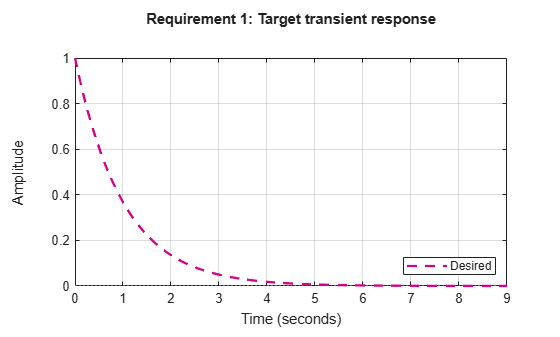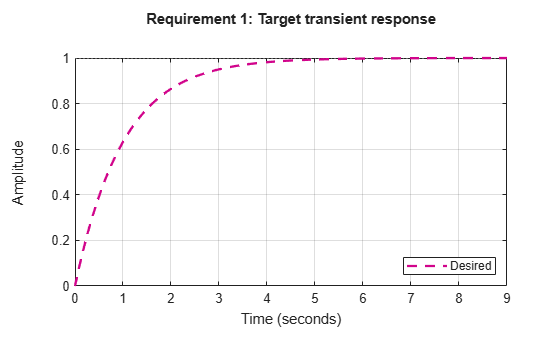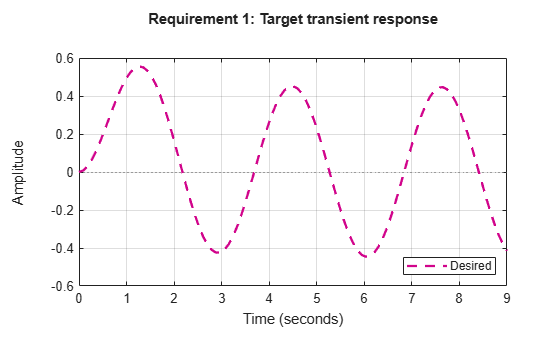# TuningGoal.Transient class

Package: TuningGoal

Transient matching requirement for control system tuning

## Description

Use the `TuningGoal.Transient` object to constrain the transient response from specified inputs to specified outputs. This tuning goal specifies that the transient response closely match the response of a reference model. Specify the closeness of the required match using the `RelGap` property of the tuning goal (see Properties). You can constrain the response to an impulse, step, or ramp input signal. You can also constrain the response to an input signal given by the impulse response of an input filter you specify.

## Construction

`Req = TuningGoal.Transient(inputname,outputname,refsys)` requires that the impulse response from `inputname` to `outputname` closely matches the impulse response of the reference model `refsys`. Specify the closeness of the required match using the `RelGap` property of the tuning goal (see Properties). `inputname` and `outputname` can describe a SISO or MIMO response of your control system. For MIMO responses, the number of inputs must equal the number of outputs.

`Req = TuningGoal.Transient(inputname,outputname,refsys,inputtype)` specifies whether the input signal that generates the constrained transient response is and impulse, step, or ramp signal.

`Req = TuningGoal.Transient(inputname,outputname,refsys,inputfilter)` specifies the input signal for generating the transient response that the tuning goal constrains. Specify the input signal as a SISO transfer function, `inputfilter`, that is the Laplace transform of the desired time-domain input signal. The impulse response of `inputfilter` is the desired input signal.

### Input Arguments

 `inputname` Input signals for the tuning goal, specified as a character vector or, for multiple-input tuning goals, a cell array of character vectors. If you are using the tuning goal to tune a Simulink® model of a control system, then `inputname` can include:Any model input.Any linear analysis point marked in the model.Any linear analysis point in an `slTuner` (Simulink Control Design) interface associated with the Simulink model. Use `addPoint` (Simulink Control Design) to add analysis points to the `slTuner` interface. Use `getPoints` (Simulink Control Design) to get the list of analysis points available in an `slTuner` interface to your model. For example, suppose that the `slTuner` interface contains analysis points `u1` and `u2`. Use `'u1'` to designate that point as an input signal when creating tuning goals. Use `{'u1','u2'}` to designate a two-channel input. If you are using the tuning goal to tune a generalized state-space (`genss`) model of a control system, then `inputname` can include: Any input of the `genss` model Any `AnalysisPoint` location in the control system modelFor example, if you are tuning a control system model, `T`, then `inputname` can be any input name in `T.InputName`. Also, if `T` contains an `AnalysisPoint` block with a location named `AP_u`, then `inputname` can include `'AP_u'`. Use `getPoints` to get a list of analysis points available in a `genss` model.If `inputname` is an `AnalysisPoint` location of a generalized model, the input signal for the tuning goal is the implied input associated with the `AnalysisPoint` block:For more information about analysis points in control system models, see Mark Signals of Interest for Control System Analysis and Design. `outputname` Output signals for the tuning goal, specified as a character vector or, for multiple-output tuning goals, a cell array of character vectors. If you are using the tuning goal to tune a Simulink model of a control system, then `outputname` can include:Any model output.Any linear analysis point marked in the model.Any linear analysis point in an `slTuner` (Simulink Control Design) interface associated with the Simulink model. Use `addPoint` (Simulink Control Design) to add analysis points to the `slTuner` interface. Use `getPoints` (Simulink Control Design) to get the list of analysis points available in an `slTuner` interface to your model. For example, suppose that the `slTuner` interface contains analysis points `y1` and `y2`. Use `'y1'` to designate that point as an output signal when creating tuning goals. Use `{'y1','y2'}` to designate a two-channel output. If you are using the tuning goal to tune a generalized state-space (`genss`) model of a control system, then `outputname` can include: Any output of the `genss` model Any `AnalysisPoint` location in the control system modelFor example, if you are tuning a control system model, `T`, then `outputname` can be any output name in `T.OutputName`. Also, if `T` contains an `AnalysisPoint` block with a location named `AP_u`, then `outputname` can include `'AP_u'`. Use `getPoints` to get a list of analysis points available in a `genss` model.If `outputname` is an `AnalysisPoint` location of a generalized model, the output signal for the tuning goal is the implied output associated with the `AnalysisPoint` block:For more information about analysis points in control system models, see Mark Signals of Interest for Control System Analysis and Design. `refsys` Reference system for target transient response, specified as a dynamic system model, such as a `tf`, `zpk`, or `ss` model. The desired transient response is the response of this model to the input signal specified by `inputtype` or `inputfilter`. The reference model must be stable, and the series connection of the reference model with the input shaping filter must have no feedthrough term. `inputtype` Type of input signal that generates the constrained transient response, specified as one of the following values: `'impulse'` — Constrain the response at `outputname` to a unit impulse applied at `inputname`.`'step'` — Constrain the response to a unit step. Using `'step'` is equivalent to using the `TuningGoal.StepTracking` design goal. `'ramp'` — Constrain the response to a unit ramp, `u = t`. Default: `'impulse'` `inputfilter` Custom input signal for generating the transient response, specified as a SISO transfer function (`tf` or `zpk`) model that represents the Laplace transform of the desired input signal. `inputfilter` must be continuous, and can have no poles in the open right-half plane. The frequency response of `inputfilter` gives the signal spectrum of the desired input signal, and the impulse response of `inputfilter` is the time-domain input signal. For example, to constrain the transient response to a unit-amplitude sine wave of frequency `w`, set `inputfilter` to `tf(w,[1,0,w^2])`. This transfer function is the Laplace transform of sin(wt). The series connection of `refsys` with `inputfilter` must have no feedthrough term.

## Properties

`ReferenceModel`

Reference system for target transient response, specified as a SISO or MIMO state-space (`ss`) model. When you use the tuning goal to tune a control system, the transient response from `inputname` to `outputname` is tuned to match this target response to within the tolerance specified by the `RelGap` property.

The `refsys` argument to `TuningGoal.Transient` sets the value of `ReferenceModel` to `ss(refsys)`.

`InputShaping`

Input signal for generating the transient response, specified as a SISO `zpk` model that represents the Laplace transform of the time-domain input signal. `InputShaping` must be continuous, and can have no poles in the open right-half plane. The value of this property is populated using the `inputtype` or `inputfilter` arguments used when creating the tuning goal.

For tuning goals created using the `inputtype` argument, `InputShaping` takes the following values:

`inputtype``InputShaping`
`'impulse'`1
`'step'`1/s
`'ramp'`1/s2

For tuning goals created using an `inputfilter` transfer function, `InputShaping` takes the value `zpk(inputfilter)`.

The series connection of `ReferenceModel` with `InputShaping` must have no feedthrough term.

Default: 1

`RelGap`

Maximum relative matching error, specified as a positive scalar value. This property specifies the matching tolerance as the maximum relative gap between the target and actual transient responses. The relative gap is defined as:

`$\text{gap}=\frac{{‖y\left(t\right)-{y}_{ref}\left(t\right)‖}_{2}}{{‖{y}_{ref\left(tr\right)}\left(t\right)‖}_{2}}.$`

y(t) – yref(t) is the response mismatch, and 1 – yref(tr)(t) is the transient portion of yref (deviation from steady-state value or trajectory). ${‖\text{\hspace{0.17em}}\cdot \text{\hspace{0.17em}}‖}_{2}$ denotes the signal energy (2-norm). The gap can be understood as the ratio of the root-mean-square (RMS) of the mismatch to the RMS of the reference transient

Increase the value of `RelGap` to loosen the matching tolerance.

Default: 0.1

`InputScaling`

Input signal scaling, specified as a vector of positive real values.

Use this property to specify the relative amplitude of each entry in vector-valued input signals when the choice of units results in a mix of small and large signals. This information is used to scale the closed-loop transfer function from `Input` to `Output` when the tuning goal is evaluated.

Suppose T(s) is the closed-loop transfer function from `Input` to `Output`. The tuning goal is evaluated for the scaled transfer function Do–1T(s)Di. The diagonal matrices Do and Di have the `OutputScaling` and `InputScaling` values on the diagonal, respectively.

The default value, `[]` , means no scaling.

Default: `[]`

`OutputScaling`

Output signal scaling, specified as a vector of positive real values.

Use this property to specify the relative amplitude of each entry in vector-valued output signals when the choice of units results in a mix of small and large signals. This information is used to scale the closed-loop transfer function from `Input` to `Output` when the tuning goal is evaluated.

Suppose T(s) is the closed-loop transfer function from `Input` to `Output`. The tuning goal is evaluated for the scaled transfer function Do–1T(s)Di. The diagonal matrices Do and Di have the `OutputScaling` and `InputScaling` values on the diagonal, respectively.

The default value, `[]` , means no scaling.

Default: `[]`

`Input`

Input signal names, specified as a as a cell array of character vectors that indicate the inputs for the transient responses that the tuning goal constrains. The initial value of the `Input` property is populated by the `inputname` argument when you create the tuning goal.

`Output`

Output signal names, specified as a cell array of character vectors that indicate the outputs where transient responses that the tuning goal constrains are measured. The initial value of the `Output` property is populated by the `outputname` argument when you create the tuning goal.

`Models`

Models to which the tuning goal applies, specified as a vector of indices.

Use the `Models` property when tuning an array of control system models with `systune`, to enforce a tuning goal for a subset of models in the array. For example, suppose you want to apply the tuning goal, `Req`, to the second, third, and fourth models in a model array passed to `systune`. To restrict enforcement of the tuning goal, use the following command:

`Req.Models = 2:4;`

When `Models = NaN`, the tuning goal applies to all models.

Default: `NaN`

`Openings`

Feedback loops to open when evaluating the tuning goal, specified as a cell array of character vectors that identify loop-opening locations. The tuning goal is evaluated against the open-loop configuration created by opening feedback loops at the locations you identify.

If you are using the tuning goal to tune a Simulink model of a control system, then `Openings` can include any linear analysis point marked in the model, or any linear analysis point in an `slTuner` (Simulink Control Design) interface associated with the Simulink model. Use `addPoint` (Simulink Control Design) to add analysis points and loop openings to the `slTuner` interface. Use `getPoints` (Simulink Control Design) to get the list of analysis points available in an `slTuner` interface to your model.

If you are using the tuning goal to tune a generalized state-space (`genss`) model of a control system, then `Openings` can include any `AnalysisPoint` location in the control system model. Use `getPoints` to get the list of analysis points available in the `genss` model.

For example, if `Openings = {'u1','u2'}`, then the tuning goal is evaluated with loops open at analysis points `u1` and `u2`.

Default: `{}`

`Name`

Name of the tuning goal, specified as a character vector.

For example, if `Req` is a tuning goal:

`Req.Name = 'LoopReq';`

Default: `[]`

## Examples

### Transient Response Requirement with Specified Input Type and Tolerance

Create a requirement for the transient response from a signal named `'r'` to a signal named `'u'`. Constrain the impulse response to match the response of transfer function $refsys=1/\left(s+1\right)$, but allow 20% relative variation between the target and tuned responses.

```refsys = tf(1,[1 1]); Req1 = TuningGoal.Transient('r','u',refsys);```

When you do not specify a response type, the requirement constrains the transient response. By default, the requirement allows a relative gap of 0.1 between the target and tuned responses. To change the relative gap to 20%, set the `RelGap` property of the requirement.

`Req1.RelGap = 0.2;`

Examine the requirement.

`viewGoal(Req1)`The dashed line shows the target impulse response specified by this requirement. You can use this requirement to tune a control system model, `T`, that contains valid input and output locations named `'r'` and `'u'`. If you do so, the command `viewGoal(Req1,T)` plots the achieved impulse response from `'r'` to `'u'` for comparison to the target response.

Create a requirement that constrains the response to a step input, instead of the impulse response.

`Req2 = TuningGoal.Transient('r','u',refsys,'step');`

Examine this requirement.

`viewGoal(Req2)``Req2` is equivalent to the following step tracking requirement:

`Req3 = TuningGoal.StepTracking('r','u',refsys);`

### Constrain Transient Response to Custom Input Signal

Create a requirement for the transient response from `'r'` to `'u'`. Constrain the response to a sinusoidal input signal, rather than to an input, step, or ramp.

To specify a custom input signal, set the input filter to the Laplace transform of the desired signal. For example, suppose you want to constrain the response to a signal of $\mathrm{sin}\omega t$. The Laplace transform of this signal is given by:

`$inputfilter=\frac{\omega }{{s}^{2}+{\omega }^{2}}.$`

Create a requirement that constrains the response at `'u'` to a sinusoidal input of natural frequency 2 rad/s at `'r'`. The response should match that of the reference system $refsys=1/\left(s+1\right)$.

```refsys = tf(1,[1 1]); w = 2; inputfilter = tf(w,[1 0 w^2]); Req = TuningGoal.Transient('u','r',refsys,inputfilter);```

Examine the requirement to see the shape of the target response.

`viewGoal(Req)`### Transient Response Goal with Limited Model Application and Additional Loop Openings

Create a tuning goal that constrains the impulse response. Set the `Models` and `Openings` properties to further configure the tuning goal’s applicability.

```refsys = tf(1,[1 1]); Req = TuningGoal.Transient('r','u',refsys); Req.Models = [2 3]; Req.Openings = 'OuterLoop'```

When tuning a control system that has an input (or analysis point) `'r'`, an output (or analysis point) `'u'`, and another analysis point at location `'OuterLoop'`, you can use `Req` as an input to `looptune` or `systune`. Setting the `Openings` property specifies that the impulse response from `'r'` to `'y'` is computed with the loop opened at `'OuterLoop'`. When tuning an array of control system models, setting the `Models` property restricts how the tuning goal is applied. In this example, the tuning goal applies only to the second and third models in an array.

## Tips

• When you use this tuning goal to tune a continuous-time control system, `systune` attempts to enforce zero feedthrough (D = 0) on the transfer that the tuning goal constrains. Zero feedthrough is imposed because the H2 norm, and therefore the value of the tuning goal (see Algorithms), is infinite for continuous-time systems with nonzero feedthrough.

`systune` enforces zero feedthrough by fixing to zero all tunable parameters that contribute to the feedthrough term. `systune` returns an error when fixing these tunable parameters is insufficient to enforce zero feedthrough. In such cases, you must modify the tuning goal or the control structure, or manually fix some tunable parameters of your system to values that eliminate the feedthrough term.

When the constrained transfer function has several tunable blocks in series, the software’s approach of zeroing all parameters that contribute to the overall feedthrough might be conservative. In that case, it is sufficient to zero the feedthrough term of one of the blocks. If you want to control which block has feedthrough fixed to zero, you can manually fix the feedthrough of the tuned block of your choice.

To fix parameters of tunable blocks to specified values, use the `Value` and `Free` properties of the block parametrization. For example, consider a tuned state-space block:

`C = tunableSS('C',1,2,3);`

To enforce zero feedthrough on this block, set its D matrix value to zero, and fix the parameter.

```C.D.Value = 0; C.D.Free = false;```

For more information on fixing parameter values, see the Control Design Block reference pages, such as `tunableSS`.

• This tuning goal imposes an implicit stability constraint on the closed-loop transfer function from `Input` to `Output`, evaluated with loops opened at the points identified in `Openings`. The dynamics affected by this implicit constraint are the stabilized dynamics for this tuning goal. The `MinDecay` and `MaxRadius` options of `systuneOptions` control the bounds on these implicitly constrained dynamics. If the optimization fails to meet the default bounds, or if the default bounds conflict with other requirements, use `systuneOptions` to change these defaults.

## Algorithms

When you tune a control system using a `TuningGoal`, the software converts the tuning goal into a normalized scalar value f(x), where x is the vector of free (tunable) parameters in the control system. The software then adjusts the parameter values to minimize f(x) or to drive f(x) below 1 if the tuning goal is a hard constraint.

For `TuningGoal.Transient`, f(x) is based upon the relative gap between the tuned response and the target response:

`$\text{gap}=\frac{{‖y\left(t\right)-{y}_{ref}\left(t\right)‖}_{2}}{{‖{y}_{ref\left(tr\right)}\left(t\right)‖}_{2}}.$`

y(t) – yref(t) is the response mismatch, and 1 – yref(tr)(t) is the transient portion of yref (deviation from steady-state value or trajectory). ${‖\text{\hspace{0.17em}}\cdot \text{\hspace{0.17em}}‖}_{2}$ denotes the signal energy (2-norm). The gap can be understood as the ratio of the root-mean-square (RMS) of the mismatch to the RMS of the reference transient

## Version History

Introduced in R2016a

expand all